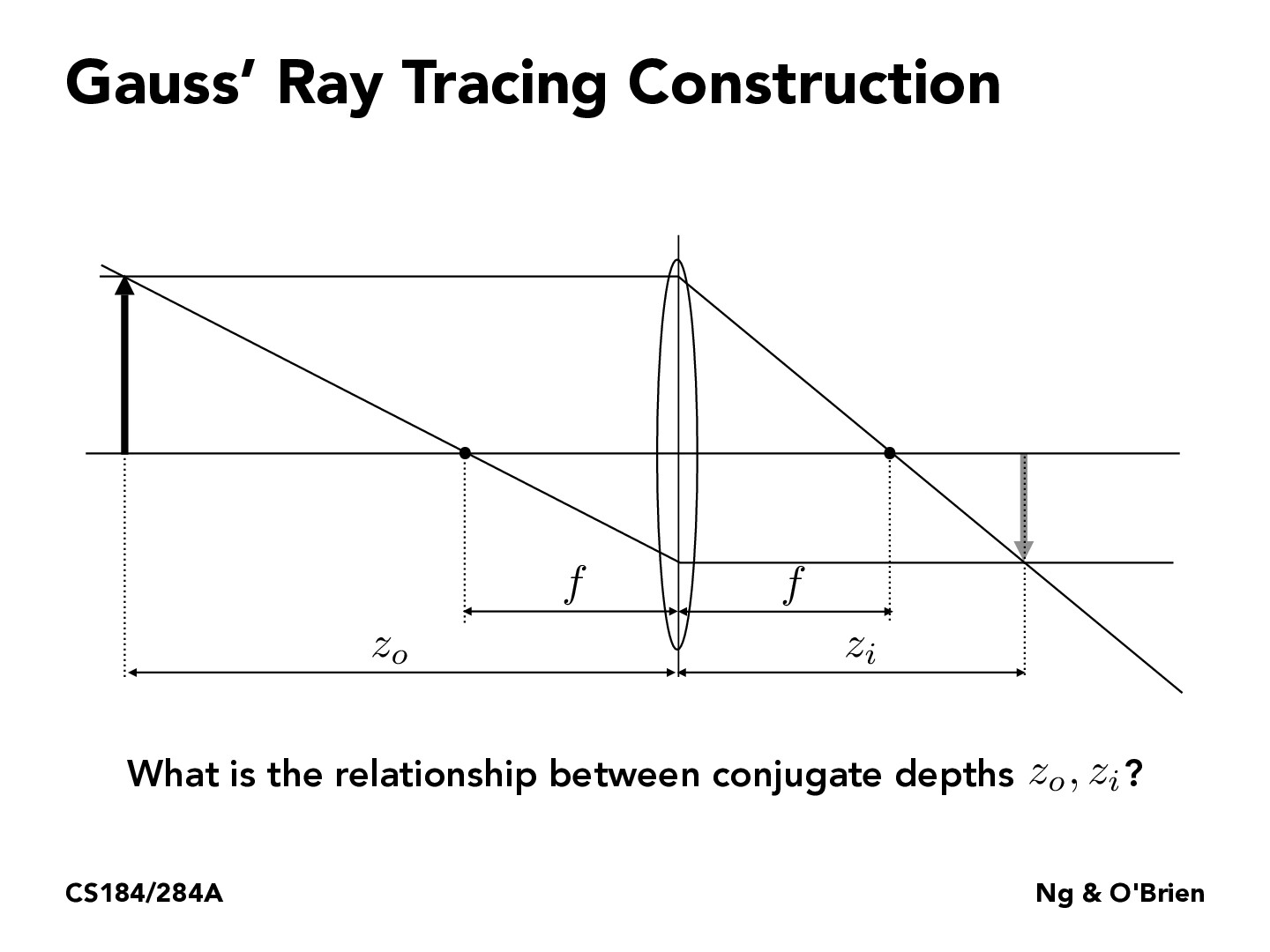Lecture 15: Cameras & Lenses (74)Sicheng-Pan

With some simple math we can derive the following formula: $\frac{1}{z_o}+\frac{1}{z_i}=\frac{1}{f}$

You must be enrolled in the course to comment